# Research interests

 geometric variational problems and calculus of variations finite- and infinite-dimensional differential geometry variational convergence of discretized problems geometric curvature functionals self-avoiding energies fractional Sobolev spaces numerical optimization optimization in Riemannian manifolds

# Publications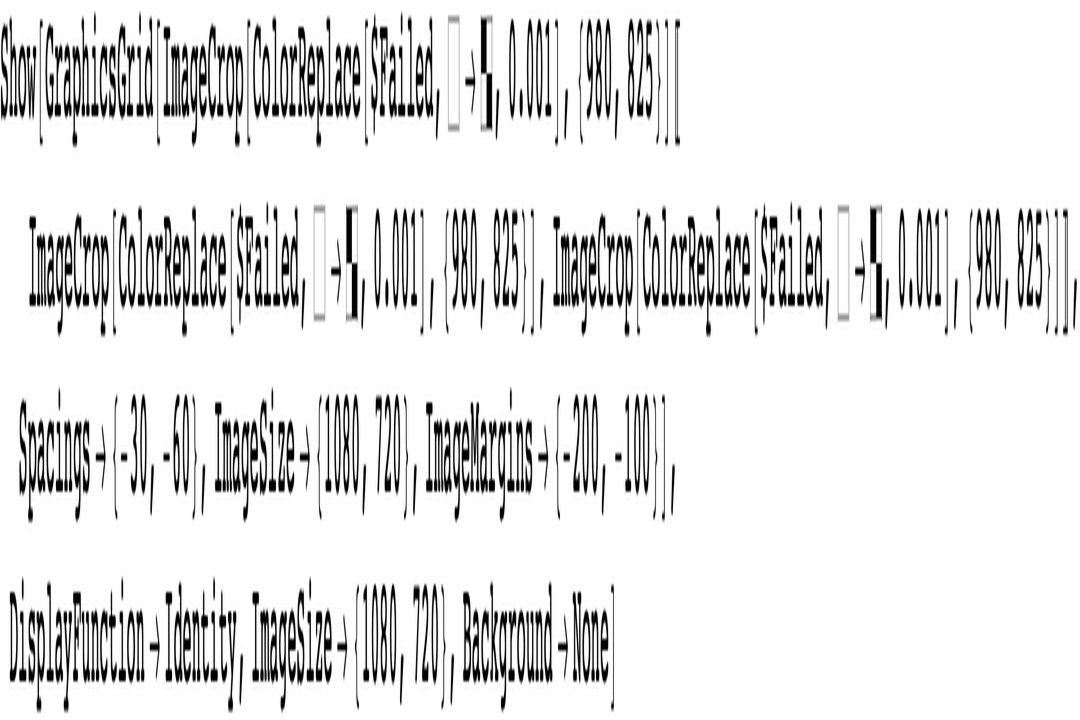A Projected Gradient Flow for the Generalized Integral Menger Curvature in the Hilbert Case Jan Knappmann, Henrik Schumacher, Daniel Steenebrügge, Heiko von der Mosel (2021) We establish long-time existence for a projected Sobolev gradient flow of generalized integral Menger curvature in the Hilbert case, and provide $C^{1,1}$-bounds in time for the solution that only depend on the initial curve. The self-avoidance property of integral Menger curvature guarantees that the knot class of the initial curve is preserved under the flow, and the projection ensures that each curve along the flow is parametrized with the same speed as the initial configuration. Finally, we describe how to simulate this flow numerically with substantially higher efficiency than in the corresponding numerical $L^2$ gradient descent or other optimization methods.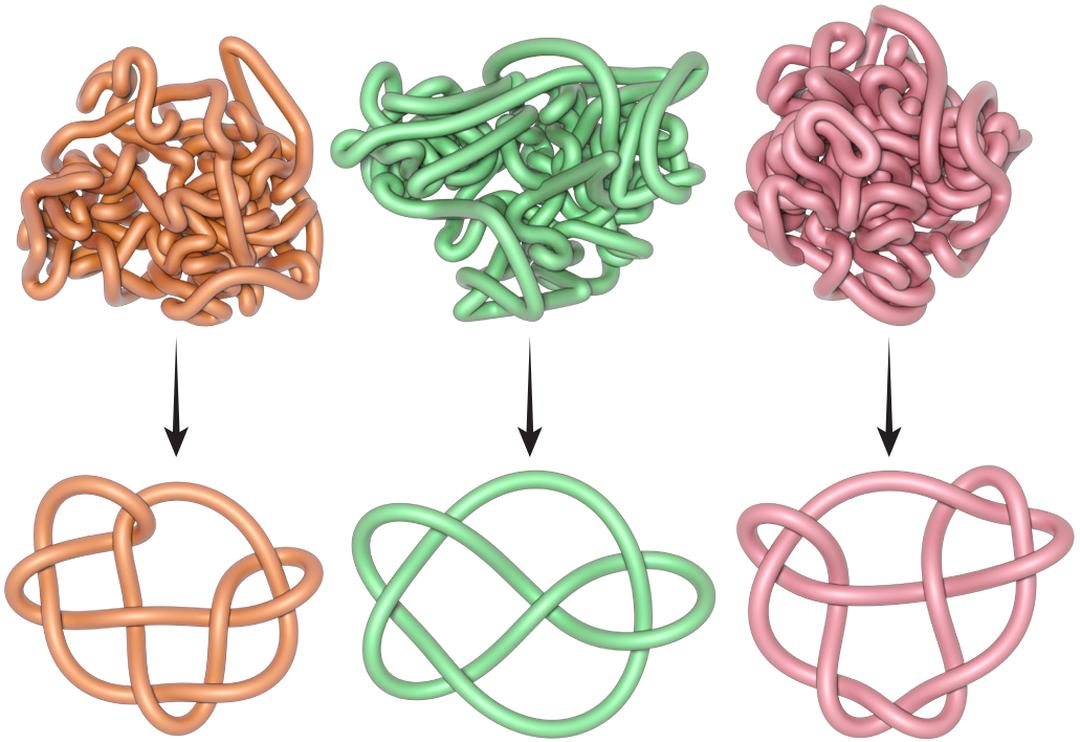Repulsive Curves Christopher Yu, Henrik Schumacher, Keenan Crane (2020) Curves play a fundamental role across computer graphics, physical simulation, and mathematical visualization, yet most tools for curve design do nothing to prevent crossings or self-intersections. This paper develops efficient algorithms for (self-)repulsion of plane and space curves that are well-suited to problems in computational design. Our starting point is the so-called tangent-point energy, which provides an infinite barrier to self-intersection. In contrast to local collision detection strategies used in, e.g., physical simulation, this energy considers interactions between all pairs of points, and is hence useful for global shape optimization: local minima tend to be aesthetically pleasing, physically valid, and nicely distributed in space. A reformulation of gradient descent, based on a Sobolev-Slobodeckij inner product enables us to make rapid progress toward local minima---independent of curve resolution. We also develop a hierarchical multigrid scheme that significantly reduces the per-step cost of optimization. The energy is easily integrated with a variety of constraints and penalties (e.g., inextensibility, or obstacle avoidance), which we use for applications including curve packing, knot untangling, graph embedding, non-crossing spline interpolation, flow visualization, and robotic path planning.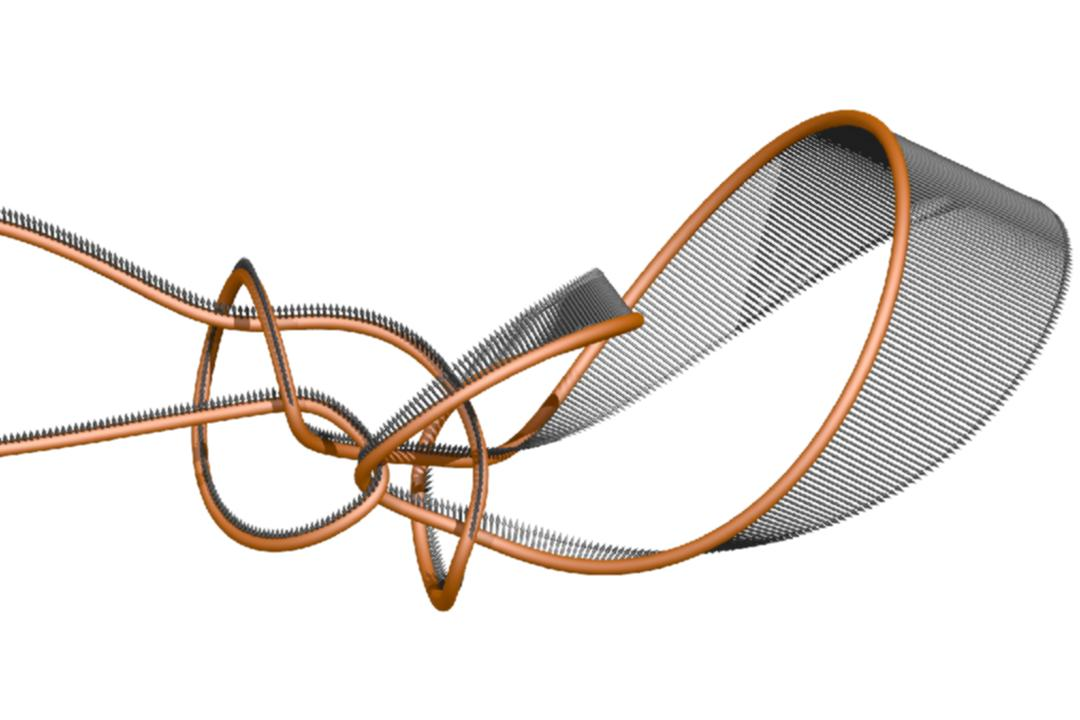Sobolev Gradients for the Möbius Energy Philipp Reiter, Henrik Schumacher (2020) Aiming at optimizing the shape of closed embedded curves within prescribed isotopy classes, we use a gradient-based approach to approximate stationary points of the Möbius energy. The gradients are computed with respect to Sobolev inner products similar to the $W^{3/2,2}$-inner product. This leads to optimization methods that are significantly more efficient and robust than standard techniques based on $L^2$-gradients.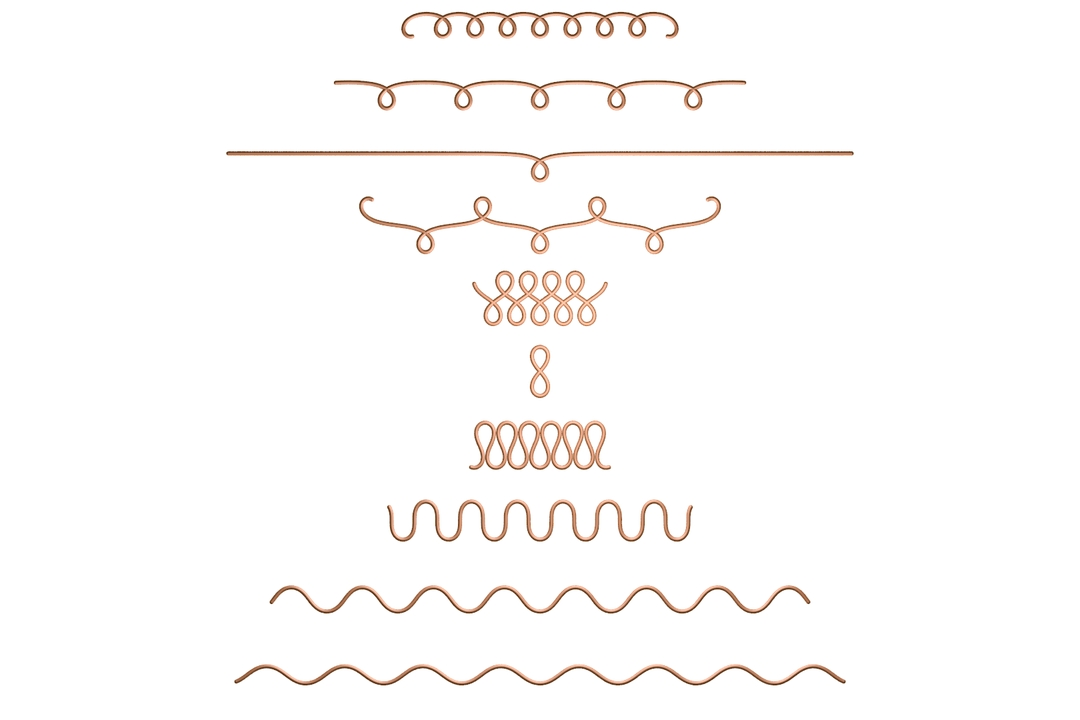Variational Methods for Discrete Geometric Functionals Henrik Schumacher, Max Wardetzky (2020) While consistent discrete notions of curvatures and differential operators have been widely studied, the question of whether the resulting minimizers converge to their smooth counterparts still remains open for various geometric functionals. Building on tools from variational analysis, and in particular using the notion of Kuratowski convergence, we offer a general framework for treating convergence of minimizers of (discrete) geometric functionals. We show how to apply the resulting machinery to minimal surfaces and Euler elasticae.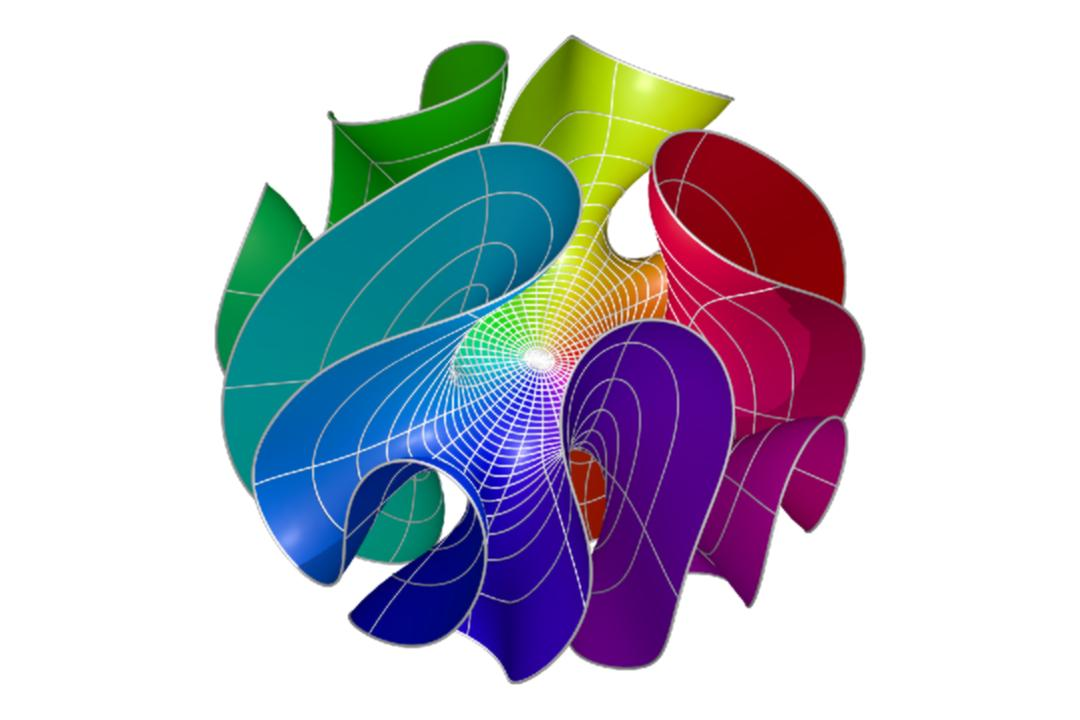Computing the conformal barycenter Jason Cantarella, Henrik Schumacher (2020) The conformal barycenter of a point cloud on the sphere at infinity of the Poincaré ball model of hyperbolic space is a hyperbolic analogue of the geometric median of a point cloud in Euclidean space. It was defined by Douady and Earle as part of a construction of a conformally natural way to extend homeomorphisms of the circle to homeomorphisms of the disk, and it plays a central role in Millson and Kapovich's model of the configuration space of cyclic linkages with fixed edge lengths. In this paper we consider the problem of computing the conformal barycenter. Abikoff and Ye have given an iterative algorithm for measures on $\mathbb{S}^d$ which is guaranteed to converge. We analyze Riemannian versions of Newton's method computed in the intrinsic geometry of the Poincare ball model. We give Newton-Kantorovich (NK) conditions under which we show that Newton's method with fixed step size is guaranteed to converge quadratically to the conformal barycenter for measures on any $\mathbb{S}^d$ (including infinite-dimensional spheres). For measures given by $n$ atoms on a finite dimensional sphere which obey the NK conditions, we give an explicit linear bound on the computation time required to approximate the conformal barycenter to fixed error. We prove that our NK conditions hold for all but exponentially few $n$ atom measures. For all measures with a unique conformal barycenter we show that a regularized Newton's method with line search will always converge (eventually superlinearly) to the conformal barycenter. Though we do not have hard time bounds for this algorithm, experiments show that it is extremely efficient in practice and in particular much faster than the Abikoff-Ye iteration.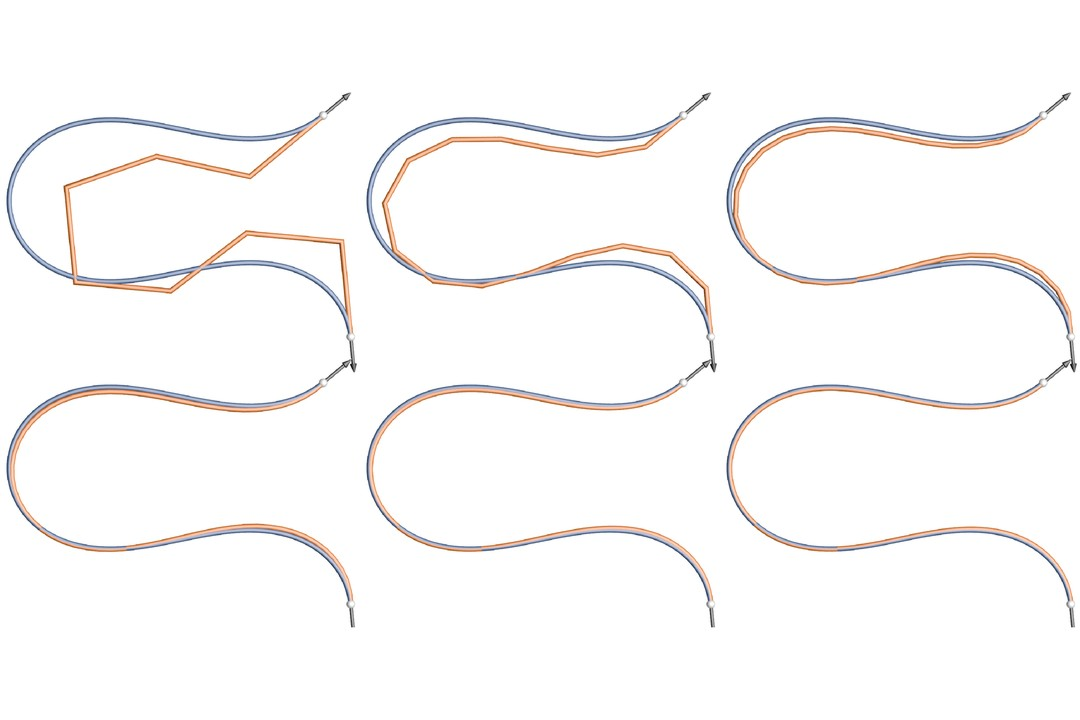Variational convergence of discrete elasticae Sebastian Scholtes, Henrik Schumacher, Max Wardetzky (2020) We discuss a discretization of the Euler--Bernoulli bending energy and of Euler elasticae under clamped boundary conditions by polygonal lines. We show Hausdorff convergence of the set of almost minimizers of the discrete bending energy to the set of smooth Euler elasticae under mesh refinement in (i) the $W^{1,\infty}$-topology for piecewise-linear interpolation; and in (ii) the $W^{2,p}$-topology, $p \in [2,\infty [$, using a suitable smoothing operator to create $W^{2,p}$-curves from polygons.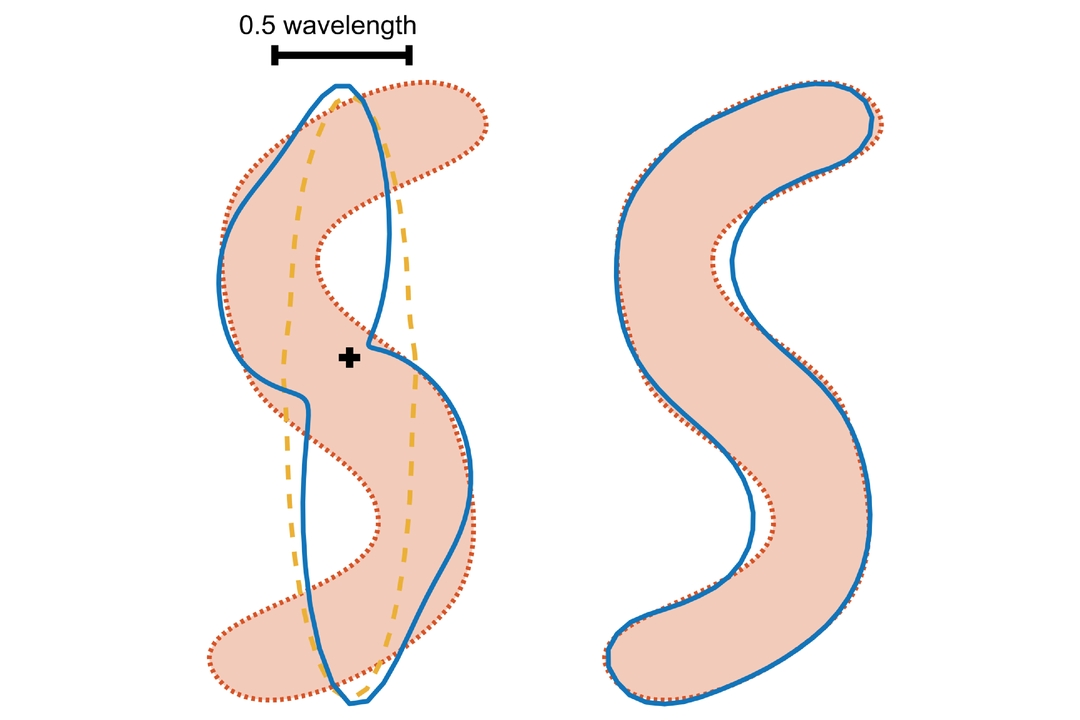Elastic energy regularization for inverse obstacle scattering problems J. Eckhardt, R. Hiptmair, T. Hohage, H. Schumacher, M. Wardetzky (2019) This paper is motivated by inverse problems in which the boundary curve of a smooth bounded domain has to be reconstructed from indirect measurements. As a classical example we study acoustic inverse obstacle scattering problems for cylindrical sound-soft scatterers using far-field measurements of scattered time-harmonic waves. By introducing a shape manifold as a solution set we allow the reconstruction of general, not necessarily star-shaped, curves. The bending energy is used as a stabilizing term in Tikhonov regularization to gain independence of the parametrization. Moreover, we discuss how self-intersections can be avoided by penalization with the Möbius energy and prove the regularizing property of our approach as well as convergence rates under variational source conditions. In a second part of the paper a discrete setting is introduced, and we describe a numerical method for finding the minimizer of the Tikhonov functional on the shape-manifold. Numerical examples demonstrate that our method can reconstruct non-star-shaped obstacles.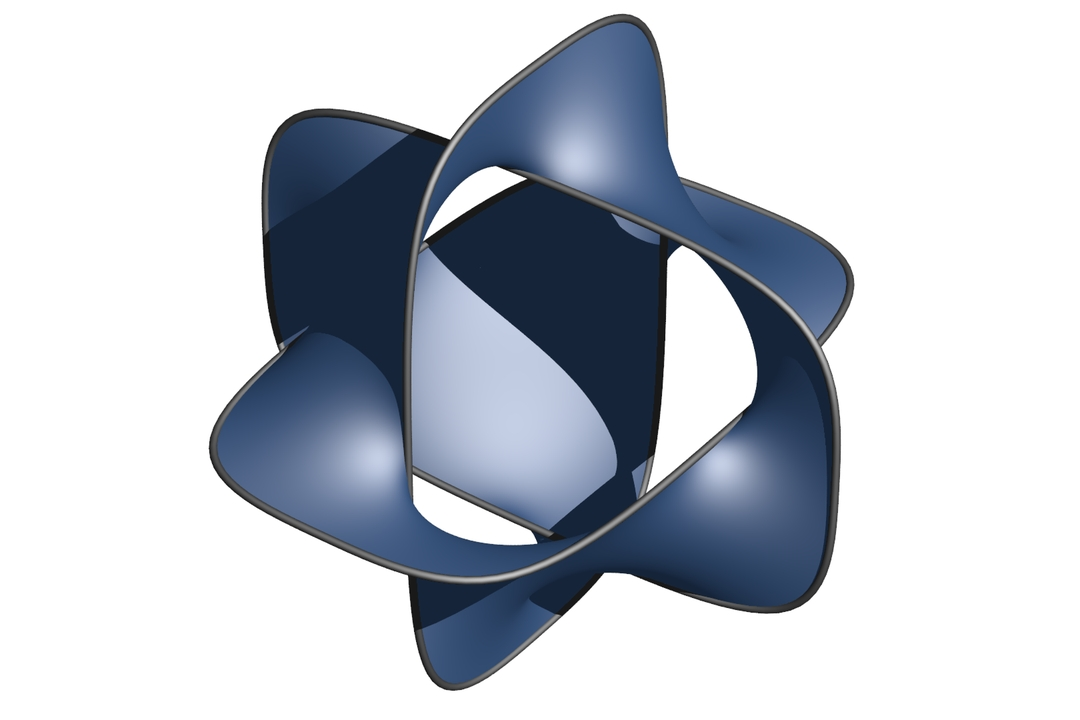Variational convergence of discrete minimal surfaces Henrik Schumacher, Max Wardetzky (2019) Building on and extending tools from variational analysis and relying on certain a~priori assumptions, we prove Kuratowski convergence of sets of simplicial area minimizers to minimizers of the smooth Douglas-Plateau problem under simplicial refinement. This convergence is with respect to a topology that is finer than the topology of uniform convergence of both positions and surface normals.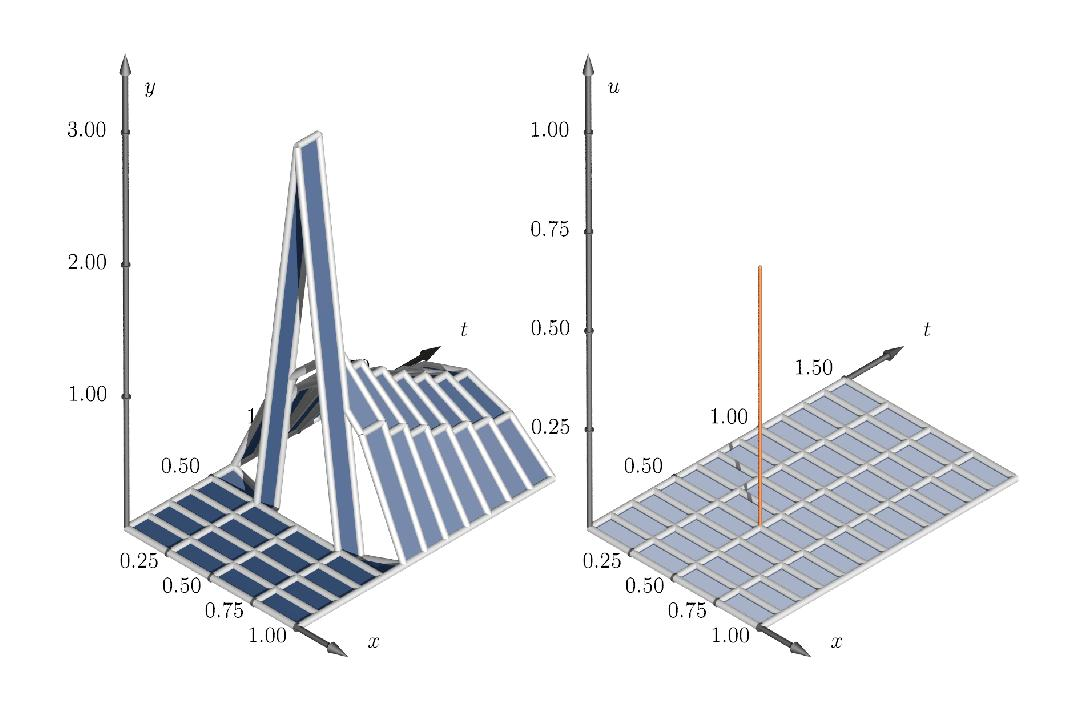Maximal discrete sparsity in parabolic optimal control with measures Evelyn Herberg, Michael Hinze, Henrik Schumacher (2019) We consider variational discretization of a parabolic optimal control problem governed by space-time measure controls. For the state discretization we use a Petrov-Galerkin method employing piecewise constant states and piecewise linear and continuous test functions in time. For the space discretization we use piecewise linear and continuous functions. As a result the controls are composed of Dirac measures in space-time, centered at points on the discrete space-time grid. We prove that the optimal discrete states and controls converge strongly in $L^q$ and weakly-$*$ in $\mathcal{M}$, respectively, to their smooth counterparts, where $q \in (1,\min\{2,1+2/d\}]$ is the spatial dimension. Furthermore, we compare our approach to [Casas, Kunisch - Parabolic control problems in space-time measure spaces], where the corresponding control problem is discretized employing a discontinuous Galerkin method for the state discretization and where the discrete controls are piecewise constant in time and Dirac measures in space. Numerical experiments highlight the features of our discrete approach.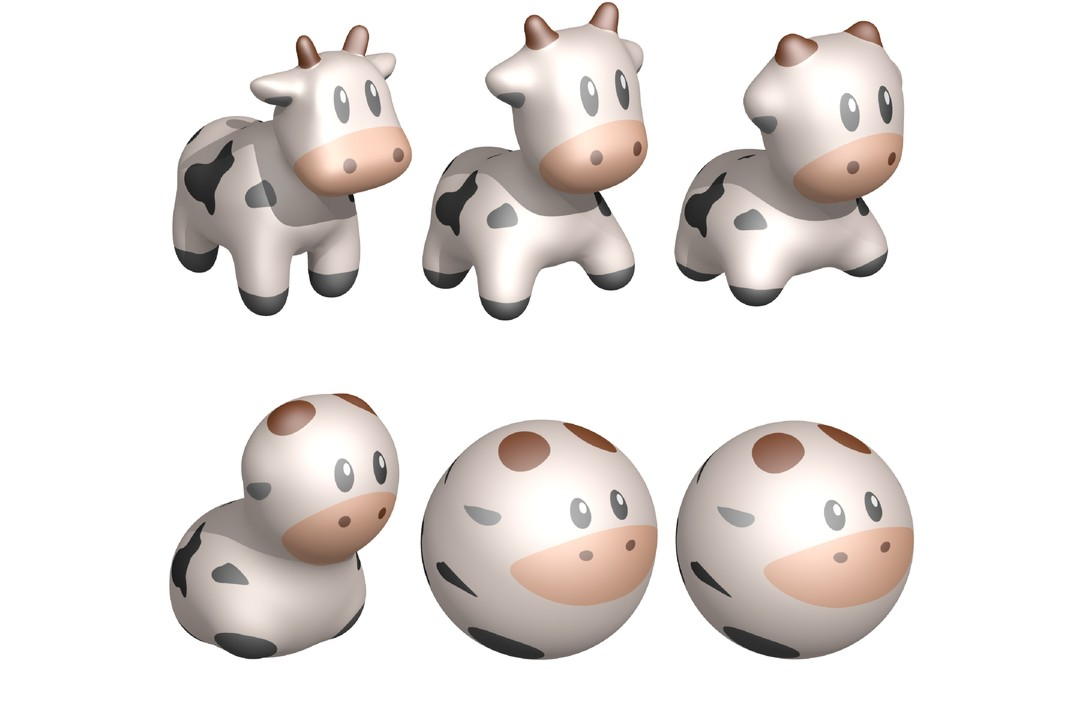Pseudogradient flows of geometric energies Henrik Schumacher (2018) For differentiable functions on Riemannian manifolds, the gradient vector field and its induced gradient flow are well-defined and well-understood concepts. Unfortunately, many nonquadratic, infinite-dimensional optimization problems cannot be formulated on Riemannian manifolds in a convenient way. We introduce a category of infinite-dimensional Banach manifolds that allows us to define a generalized gradient. Moreover, we show short-time existence of the induced flows and apply their discretizations to the numerical minimization of various geometric energies of immersed curves and surfaces.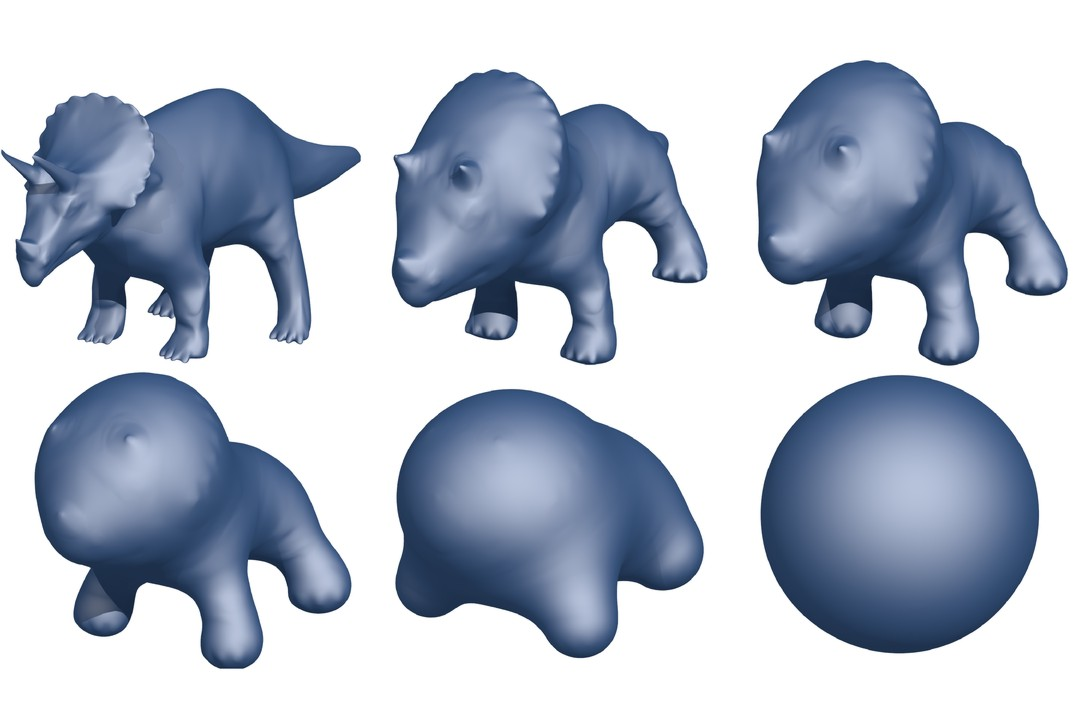On $H^2$-gradient Flows for the Willmore Energy Henrik Schumacher (2017) We show that the concept of $H^2$-gradient flow for the Willmore energy and other functionals that depend at most quadratically on the second fundamental form is well-defined in the space of immersions of Sobolev class $W^{2,p}$ from a compact, $n$-dimensional manifold into Euclidean space, provided that $p \geq 2$ and $p>n$. We also discuss why this is not true for Sobolev class $H^2= W^{2,2}$. In the case of equality constraints, we provide sufficient conditions for the existence of the projected $H^2$-gradient flow and demonstrate its usability for optimization with several numerical examples.

# Research ReportsPolyhedral discretizations of tangent-point energies Henrik Schumacher (2021) We discuss some of our recent findings on the consistency error of polyehdral discretizations of tangent-point energies for curves and surfaces. Moreover, we outline how Barnes-Hut and fast multipole techniques can be used to devise more efficient discretization.Convergence of discrete elastica Sebastian Scholtes, Henrik Schumacher, Max Wardetzky (2012) Using techniques related to the notions of epigraph distance and Attouch-Wets-convergence, we show that under appropriate boundary conditions discrete elastica (i.e., polygonal curves of some fixed length that minimize a certain discrete bending energy) converge to smooth elastica (i.e., smooth curves of some given length that minimize smooth bending energy).

# DissertationVariational Convergence and Discrete Minimal Surfaces Henrik Schumacher (2015) This work is concerned with the convergence behavior of the solutions to parametric variational problems. An emphasis is put on sequences of variational problems that arise as discretizations of either infinite-dimensional optimization problems or infinite-dimensional operator problems. Finally, the results are applied to discretizations of the Douglas-Plateau problem and of a boundary value problem in nonlinear elasticity.